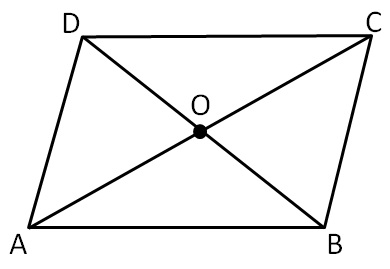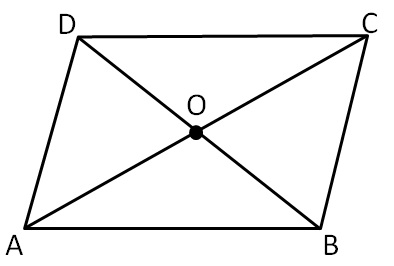both pairs of opposite sides are parallel

i.e. AB ∥ DC and AD ∥ BC

Properties of Parallelogram:

1. Opposite sides are equal
AB = CD & AD = BC

2. Opposite angles are equal
∠A = ∠C and ∠B = ∠D

∠A + ∠B = 180°

∠A + ∠D = 180°

∠C + ∠D = 180°

∠B + ∠C = 180°4. Diagonals bisect each other

AO = CO and BO = DO

Proof : Class 9 th Chapter 8 Theorem 8.6

5.Diagonals divides the Parallelogram into two congruent triangles

∆ ABC ≅ ∆ CDA and

Proof : Class 9 th Chapter 8 Theorem 8.1

1. Chapter 4 Class 8 Practical Geometry
2. Concept wise
3. Constructing Parallelogram